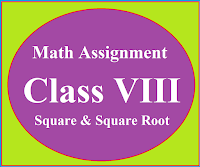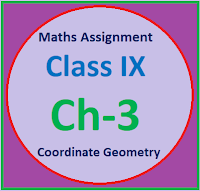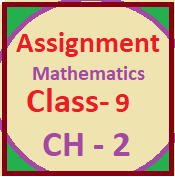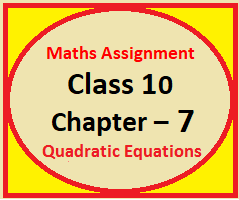## Posts

Showing posts from May, 2022

### Math Assignment Class VIII | Square & Square RootMath Assignment  Class VIII | Square & Square Root Download or Print free  assignment with answer key  for   Class  8 Squares and  Square Roots.   Important and extra questions that cover all topics of square and square root and is useful and helpful for the students. Math Assignment  Class VIII | Square & Square Root LEVEL -1

### Math Assignment Class IX Ch-3 | Coordinate GeometryMathematics Assignment  Class IX  Chapter 3  Coordinate Geometry Mathematics Assignment for Class IX Ch -3, Co-ordinate Geometry, strictly according to the CBSE syllabus. Math worksheet based on the topic Co-ordinate Geometry. Note:  First of all students should solve the NCERT question and then answer  the following questions. 1. What   is Cartesian coordinate system Ans: A system which involves the x-axis and y-axis on the two dimensional surface is called a cartesian coordinate system. 2. What we call the horizontal line in Cartesian coordinate system. Ans: Horizontal line is called the x - axis. 3. What we call the vertical   line in Cartesian coordinate system. Ans: Vertical Line is called the y - axis. 4. Which point is called the origin and what are its coordinates. Ans: The point of intersection of x - axis and y - axis is called the Origin. Coordinates of origin are (0,0) 5. What is the point on x – axis. Ans: Any point on the x - axis is (a, 0) 6. What is the point

### Math Assignment Class IX Ch-2 | PolynomialsMaths Assignment Class 9th  Chapter 2   Polynomials Mathematics Assignment for Class IX Ch -2, Polynomials, strictly  according to the CBSE syllabus. Math worksheet based on the topic Polynomial.

### Math Assignment Class X Ch-7 | Coordinate GeometryMath Assignment, Class X, Chapter 7 Coordinate Geometry Extra questions of chapter 7 class 10 Coordinate Geometry with answer and  hints to the difficult questions. Important and useful math assignment for the students of class 10 For better results Students should learn all the basic points of  Coordinate Geometry  Student should revise N C E R T book thoroughly with examples. Now revise this assignment. This assignment integrate the knowledge of the students. ASSIGNMENT FOR 10 STANDARD COORDINATE GEOMETRY Question 1 Find the distance between the following points:- a) (-6,7) and (-1,-5) Ans  b) (a,0) & (0,b) Ans [ ] c) ( +1,1) & (0, ) Ans [ ] d) (a + b, a - b) & (a - b, a + b) Ans [ b] Question 2 Find the distance of the following points from the origin:-  a) (5, -12)     Ans  b) (-5, 5)       Ans [5  ] c) (-4, -6)     Ans [2 ] Question 3 Find the value of k for which the following points are collinear: (a) (3,2), (4, k), (5,3)           Ans [5/2] b) (3,5), (m, 6